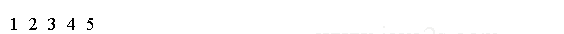There are at least two steps involved in working with a thread:

• Creating an object of the Thread class
• Invoking the start() method of the Thread class to start the thread

We can use the default constructor of the Thread class to create a Thread object.

```Thread  simplestThread = new Thread();
```

We must call its start() method to start the thread represented by that object.

```simplestThread.start();
```

There are three ways you can specify your code to be executed by a thread:

• By implementing the Runnable interface in your class
• By using the method reference to a method that takes no parameters and returns void

When we inherit class from the `Thread` class, we should override the run() method and provide the code to be executed by the thread.

```class MyThreadClass extends Thread {
@Override
public void run() {
}
}
```

```MyThreadClass  myThread = new MyThreadClass();
```

## Implementing the Runnable Interface

We can create a class that implements the java.lang.Runnable interface. Runnable is a functional interface and it is declared as follows:

```@FunctionalInterface
public interface Runnable {
void run();
}
```

From Java 8, we can use a lambda expression to create an instance of the Runnable interface.

```Runnable  aRunnableObject = () ->  System.out.println("Hello Java  thread!");
```

Create an object of the Thread class using the constructor that accepts a Runnable object.

```Thread  myThread = new Thread(aRunnableObject);
```

Start the thread by calling the start() method of the thread object.

```myThread.start();
```

The thread will execute the code contained in the body of the lambda expressions.

## Using a Method Reference

From Java 8, we can use the method reference of a method of any class that takes no parameters and returns void as the code to be executed by a thread.

The following code declares a ThreadTest class that contains an execute() method.

The method contains the code to be executed in a thread.

```public class ThreadTest {
public static void execute() {
}
}
```

The following code uses the method reference of the execute() method of the ThreadTest class to create a Runnable object:

```Thread  myThread = new Thread(ThreadTest::execute);
```

The thread will execute the code contained in the execute() method of the ThreadTest class.

The following code shows how to create a thread and print integers from 1 to 5 on the standard output.

```public class Main {
public static void main(String[] args) {
//w w w.  j  a v  a  2 s  .  co m
t.start();
}

public static void print() {
for (int i = 1; i <= 5; i++) {
System.out.print(i + "  ");
}
}
}
```

The code above generates the following result.